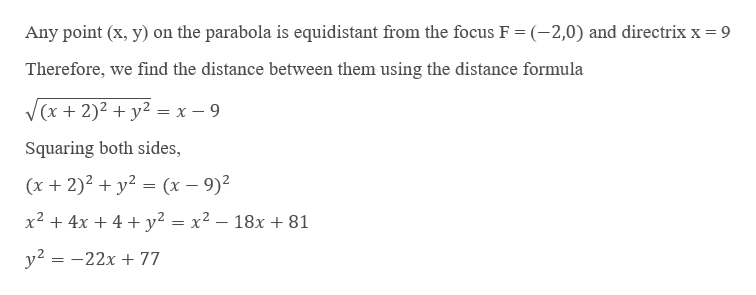# Find an equation of a parabola with focus at (−2,0) and with directrix x=9(Express your answer in the form x=f(y)

Question
46 views

Find an equation of a parabola with focus at (−2,0) and with directrix x=9

check_circle

star
star
star
star
star
1 Rating
Step 1

Given that the parabola has the following details:

Step 2

To find: Equation of parabola...help_outlineImage TranscriptioncloseAny point (x, y) on the parabola is equidistant from the focus F (-2,0) and directrix x 9 Therefore we find the distance between them using the distance formula (x2)2 y2 x - 9 Squaring both sides, (x 2)2y(x - 9)2 x24x4 y2 = x2- 18x +81 y2 22x77 fullscreen

### Want to see the full answer?

See Solution

#### Want to see this answer and more?

Solutions are written by subject experts who are available 24/7. Questions are typically answered within 1 hour.*

See Solution
*Response times may vary by subject and question.
Tagged in

### Other